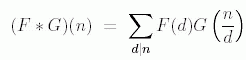ENG  RUS Timus Online JudgeOnline Judge
Problems
Authors
Online contests
Site news
Webboard
Problem set
Submit solution
Judge status
Guide
Register
Authors ranklist
Current contest
Scheduled contests
Past contests
Rules

Contest is over

## C. Multiplicative Functions

Time limit: 2.0 second
Memory limit: 64 MB
In number theory, a multiplicative function is an arithmetic function F(n) of the positive integer n with property that F(1) = 1 and whenever a and b are coprime (gcd(a, b) = 1), then F(ab) = F(a)F(b).
The function E(n) defined by E(n) = 1 if n = 1 and = 0 if n > 1, is sometimes called multiplication unit for Dirichlet convolution or simply the unit function. If F and G are two multiplicative functions, one defines a new multiplicative function F `*` G, the Dirichlet convolution of F and G, bywhere the sum extends over all positive divisors d of n. With this operation, the set of all multiplicative functions turns into an abelian group; the identity element is E.
In this task you have to find the inverse of a multiplicative function. To cope with overflow problem, we define arithmetic functions as: F: N —> Z2007 where N is the set of positive integers, and Z2007 is a residue ring (ring of integers 0–2006, where arithmetic operations + and × are performed modulo 2007). Function G is called the inverse of function F if and only if F `*` G = G `*` F = E.
You are given the first N values of function F, you need to find the first N values of the inverse function G.

### Input

In the first line of the input one number N is written (1 ≤ N ≤ 104). In the second line values F(1), F(2), F(3), …, F(N) are listed. Numbers are separated by spaces. (Each value is nonnegative and doesn't exceed 2006.)

### Output

In the first line of the output print first N values of inverse function G, separated by spaces: G(1), G(2), …, G(N).

### Sample

inputoutput
```16
1 1 1 1 1 1 1 1 1 1 1 1 1 1 1 1
```
```1 2006 2006 0 2006 1 2006 0 0 1 2006 0 2006 1 1 0
```
Problem Source: Novosibirsk SU Contest. Petrozavodsk training camp, September 2007
To submit the solution for this problem go to the Problem set: 1554. Multiplicative Functions